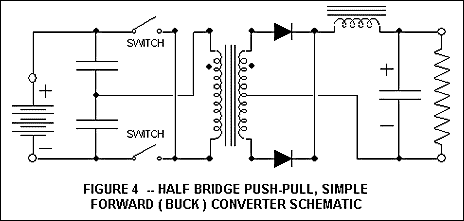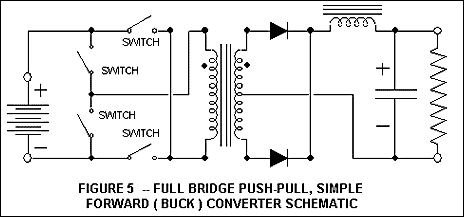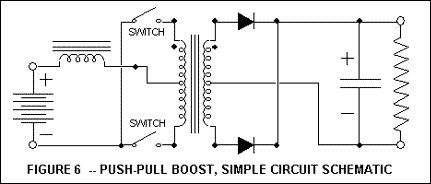# Buck Boost Transformer / Push Pull Transformer

When it comes to power conversion, the buck boost or “push pull” transformer application is well known. The buck boost transformer configuration is widely used in converting direct current (D.C.) voltage into another value of D.C. voltage, and in inverters. Inverters convert direct current into alternating current (A.C.). The push pull transformer is usually the preferred choice in high power switching transformer applications exceeding one kilowatt. It is usually used in a circuit known as a “forward converter” circuit,and it may also be referred to as an “inverter”, “D.C. converter”, “buck”, “feed forward”, and others. A basic “forward converter” transformer circuit is illustrated in Figure 1A. It is not a push pull transformer application. The output inductor reduces ripple voltage. Pulse width modulation is used to control the value of the output voltage.A center-tapped buck boost transformer application circuit is illustrated in Figure 2A. Figure 2A only shows one output. Multiple voltage outputs are possible by using either a tapped secondary winding or using multiple secondaries.*Some other buck boost transformer versions are discussed further below and illustrated in Figures 3, 4, 5, and 6. (These include some push pull transformers without the center-taps.).

The core of the transformer in Figure 1A is operated in a unipolar fashion. Unipolar operation is depicted graphically in Figure 1B. The core’s magnetic “B-H” loop remains in one quadrant of the “B-H” grid. A loop occurs once every cycle. The flux density “B” and the magnetizing force “H” never cross zero hence always retain the same (or one) polarity. “H” does not have to return to zero value. The core in a push pull transformer has bipolar operation. Both “B” and “H” cross zero value and reverse polarity. Bipolar operation is depicted graphically in Figure 2B. Note that the “dB” value (change in B) in Figure 2B for the bipolar push pull transformer can be more than twice the “dB” value shown in Figure 1B for the unipolar forward converter (assuming the same core material). Push pull transformer (bipolar) operation permits one to handle the same amount of power in a smaller package than for that of a unipolar operation. There are tradeoffs. The buck boost transformer operation requires more switching elements and its control circuitry is more complicated. Consequently a push pull transformer application is more expensive. The voltage pulses must be adequately controlled to avoid phenomena known as saturation walk. Center tapped push pull transformers have winding capacitance issues at higher frequencies. Winding imbalances can contribute to saturation walk.

Power ratings for push pull or buck boost transformer can vary from a fraction of a Watt to Kilowatts. Megawatts is possible, but definitely beyond Butler Winding’s capabilities. Size correlates with power hence size (and weight) can vary from a fraction of a cubic centimeter (several grams) to multiple cubic meters (thousands of kilograms). Buck boost transformers can be wound on toroids, bobbins, and tubes. (LINK: Core materials) vary depending on the application. Laminated or tape wound grain oriented silicon steel is common for low frequency inverter buck boost transformers. Ferrite core materials are common for high frequency switching push pull transformers. If minimal size is a requirement, nickel-iron alloys may be chosen for the 1 to 20 kilohertz range. Minimal energy storage is desired so cores have minimal air gaps in their structure.

#### Push-Pull - Buck Boost Transformer Rectification

The push pull / buck boost transformer in Figure 3 is the same as the push pull transformer in Figure 2A except for secondary rectification. Figure 2A achieves full wave rectification using a center-tap. It requires two diodes. Figure 3 achieves full wave rectification with a full wave bridge. It requires four diodes. Four diodes result in more power loss, but elimination of the center-tap simplifies transformer construction and reduces winding capacitance. The primary and secondary winding halves as shown in Figure 2A conduct current on alternate half cycles. Their maximum duty cycle is a 0.5 ratio (or 50%). Figure 3 requires approximately half of the secondary turns of Figure 2A, but its secondary winding may see a maximum duty cycle near 1 (or 100%), hence its wire must handle twice the r.m.s. current value. Both transformers are about the same size.#### Half Bridge Push-Pull Transformers

Compare figure 4 to figure 2A. Figure 4 is a half bridge push pull / buck boost transformer application.This configuration eliminates the primary center-tap and reduces primary winding capacitance. The two series connected capacitors shown in Figure 4 effectively cut the input voltage to the push pull transformer in half. Consequently, for the same power rating, the push pull / buck boost transformer requires one quarter of the total primary turns to support the halved voltage, but it must handle twice the amount of input current. The primary winding may see a maximum current duty cycle near 1, hence its wire may see 4 times the r.m.s current value as wire used in the primary winding halves of Figure 2A. Both transformers are about the same size. To achieve the same output voltage, the number of secondary turns is about the same as that of figure 2A, but the secondary over primary turns ratio is quadrupled because the primary turns of figure 4 are one quarter of that of figure 2A. The output of figure 4 is a full wave center-tap configuration. Alternately, it could be a full wave bridge configuration with approximately half the number of secondary turns.

#### Full Bridge Push-Pull Transformers

Compare figure 5 to figure 4. Figure 5 is a full bridge push pull / buck boost transformer application.Like the half bridge configuration of figure 4, this configuration eliminates the primary center-tap, reduces primary winding capacitance, & is about the same size. The two series connected capacitors are replaced by two additional transistors as shown in Figure 4. The voltage supplied to the input of the push pull transformer of figure 5 is the same as that for figure 2A. For the same power rating and source voltage, the push pull transformer of figure 5 requires half the primary turns as that of figure 2A and it must handle the same amount of input current. The primary winding of figure 5 may see a max current duty cycle near 1, hence its wire may see 2 times the r.m.s current value as wire used in the primary winding halves of Figure 2A. For the same output voltage, the number of secondary turns is about the same as that of figure 2A, but the secondary over primary turns ratio is doubled because the primary turns (fig. 5) are halved. The output of figure 5 is a full wave center-tap configuration. Alternately, it could be a full wave bridge configuration with approximately half the number of secondary turns.

#### The Boost Push-Pull Transformer Application

The prior push pull transformer applications utilize an inductor in the output circuit to reduce output voltage ripple. If there were more than one output, an inductor would be used with each output. An alternate would be to place one inductor in series with the primary center-tap of a push-pull center-tap transformer. This circuit is illustrated in Figure 6.To charge the inductor the two transistors are made to conduct at the same time. Charging current flow through both halves of the primary winding but in opposite directions resulting in magnetic cancellation of each other hence the transformer windings act as a short to ground. Opening one of the transistor switches results in current flow in only one of the primary winding halves. Alternate opening of the transistor switches results in a push-pull transformer action. Control circuitry is more complex.